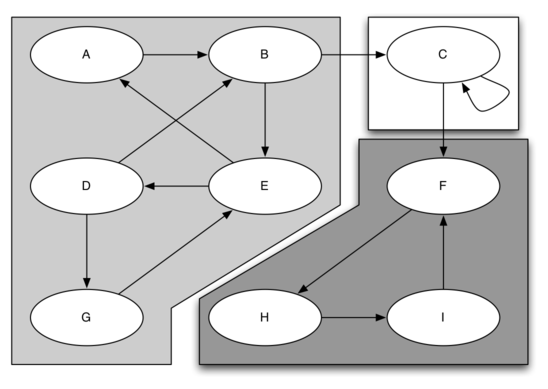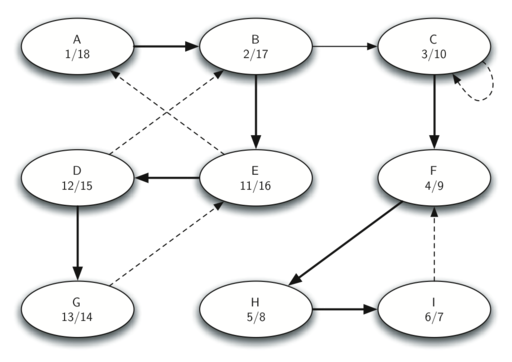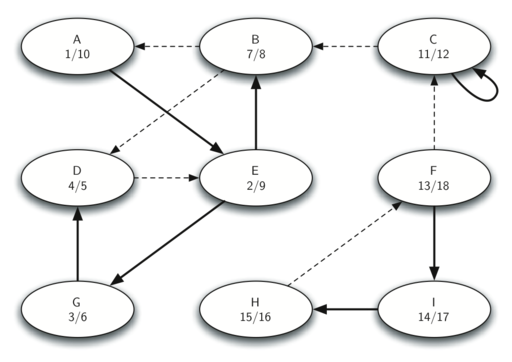# Strongly Connected Components

For the remainder of this chapter we will turn our attention to some extremely large graphs. The graphs we will use to study some additional algorithms are the graphs produced by the connections between hosts on the Internet and the links between web pages. We will begin with web pages.

Search engines like Google and Bing exploit the fact that the pages on the web form a very large directed graph. To transform the World Wide Web into a graph, we will treat a page as a vertex, and the hyperlinks on the page as edges connecting one vertex to another. The illustration below shows a very small part of the graph produced by following the links from one page to the next, beginning at Luther College’s Computer Science home page. Of course, this graph could be huge, so we have limited it to web sites that are no more than 10 links away from the CS home page.

If you study the graph above you might make some interesting observations. First you might notice that many of the other web sites on the graph are other Luther College web sites. Second, you might notice that there are several links to other colleges in Iowa. Third, you might notice that there are several links to other liberal arts colleges. You might conclude from this that there is some underlying structure to the web that clusters together web sites that are similar on some level.

One graph algorithm that can help find clusters of highly interconnected vertices in a graph is called the strongly connected components algorithm (SCC). We formally define a strongly connected component, $C$, of a graph $G$, as the largest subset of vertices $C \subset V$ such that for every pair of vertices $v, w \in C$ we have a path from $v$ to $w$ and a path from $w$ to $v$. The illustration below shows a simple graph with three strongly connected components. The strongly connected components are identified by the different shaded areas.A directed graph with three strongly connected components

Once the strongly connected components have been identified we can show a simplified view of the graph by combining all the vertices in one strongly connected component into a single larger vertex. The simplified version of the graph above is shown below.

Once again we will see that we can create a very powerful and efficient algorithm by making use of a depth first search. Before we tackle the main SCC algorithm we must look at one other definition. The transpose of a graph $G$ is defined as the graph $G^T$ where all the edges in the graph have been reversed. That is, if there is a directed edge from node A to node B in the original graph then $G^T$ will contain an edge from node B to node A. The diagrams below show a simple graph and its transposition.

Notice that the graphs above have the same two strongly connected components.

We can now describe the algorithm to compute the strongly connected components for a graph.

1. Perform a depth first search for the graph $G$ to compute the finish times for each vertex.
2. Compute $G^T$.
3. Perform a depth first search for the graph $G^T$ but in the main loop explore each vertex in decreasing order of finish time.
4. Each tree in the forest computed in step 3 is a strongly connected component. Output the vertex ids for each vertex in each tree in the forest to identify the component.

Let’s trace the operation of the steps described above on the example graph above with the three strongly connected components. Here are the start and finish times computed for the original graph:Finishing times for the original graph G

Here are the starting and finishing times computed by on the transposed graph.Finishing times for the transpose of G

Finally, the illustration below shows the forest of three trees produced in step 3 of the strongly connected component algorithm. You will notice that we do not provide you with the Python code for the SCC algorithm, we leave writing this program as an exercise.

Introduction